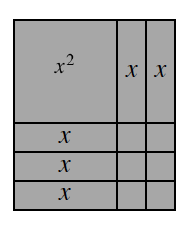### Home > CC3 > Chapter 5 > Lesson 5.2.2 > Problem5-37

5-37.Examine the rectangle formed with algebra tiles at right.

1. Find the area of the entire rectangle. That is, what is the sum of the areas of the algebra tiles?

Find the area of each of the smaller rectangles and add them together.

The area of a rectangle is its base times its height.

$x^2+5x+6$

2. Find the perimeter of the entire rectangle. Show all work.

The perimeter is the sum of the sides of a shape.

The large square is $x$ by $x$. The medium squares are $1$ by $x$. The small squares are $1$ by $1$.